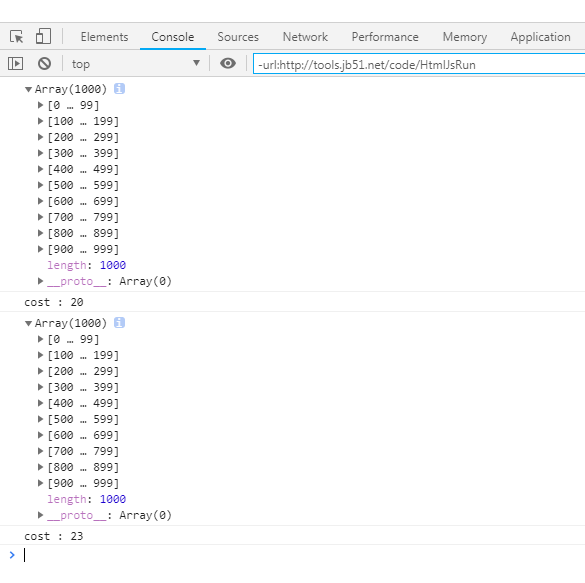JavaScript实现shuffle数组洗牌操作示例

更新时间：2019年01月03日 11:42:33   作者：司马懿字仲达我要评论

```<!DOCTYPE html PUBLIC "-//W3C//DTD XHTML 1.0 Transitional//EN"
"http://www.w3.org/TR/xhtml1/DTD/xhtml1-transitional.dtd">
<html xmlns="http://www.w3.org/1999/xhtml">
<meta http-equiv="Content-Type" content="text/html; charset=utf-8" />
<title>JavaScript shuffle数组洗牌</title>
<body>
<script>
function createArray(max) {
const arr = [];
for(let i = 0; i < max; i++) {
arr.push(i);
}
return arr;
}
function shuffleSort(arr) {
arr.sort(()=> {
//返回值大于0，表示需要交换；小于等于0表示不需要交换
return Math.random() > .5 ? -1 : 1;
});
return arr;
}
function shuffleSwap(arr) {
if(arr.length == 1) return arr;
//正向思路
//  for(let i = 0, n = arr.length; i < arr.length - 1; i++, n--) {
//    let j = i + Math.floor(Math.random() * n);
//逆向思路
let i = arr.length;
while(--i > 1) {
//Math.floor 和 parseInt 和 >>>0 和 ~~ 效果一样都是取整
let j = Math.floor(Math.random() * (i+1));
/*
//原始写法
let tmp = arr[i];
arr[i] = arr[j];
arr[j] = tmp;
*/
//es6的写法
[arr[i], arr[j]] = [arr[j], arr[i]];
}
return arr;
}
function wrap(fn, max) {
const startTime = new Date().getTime();
const arr = createArray(max);
const result = fn(arr);
const endTime = new Date().getTime();
const cost = endTime - startTime;
console.log(arr);
console.log("cost : " + cost);
}
wrap(shuffleSort, 1000);
wrap(shuffleSwap, 1000);//试验证明这种方法比第一种效率高多了
</script>
</body>
</html>

```